# Conduction: One Dimensional (Part - 3) Chemical Engineering Notes | EduRev

## Chemical Engineering : Conduction: One Dimensional (Part - 3) Chemical Engineering Notes | EduRev

The document Conduction: One Dimensional (Part - 3) Chemical Engineering Notes | EduRev is a part of the Chemical Engineering Course Heat Transfer for Engg..
All you need of Chemical Engineering at this link: Chemical Engineering

Lecture 5 - Conduction: One Dimensional, Heat Transfer

Illustration 2.3
Consider a composite wall containing 5-different materials as shown in the fig. 2.7. Calculate the rate of heat flow through the composite from the following data?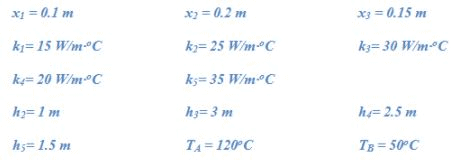Solution 2.3
Assumptions:

2. Thermal conductivity is constant for the temperature range of interest.
3. The heat loss through the edge side surface is insignificant.
4. The layers are in perfect thermal contact.
5. Area in the direction of heat flow is 1 m2.

The height of the first layer is 4 m (h1 = h2 + h).
The equivalent circuit diagram of the above composite is,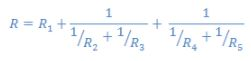On calculating equivalent resistance with the given data (Note: thickness of layer 2 = thickness of layer 3 and thickness of layer 4 = thickness of layer 5, in the heat flow direction),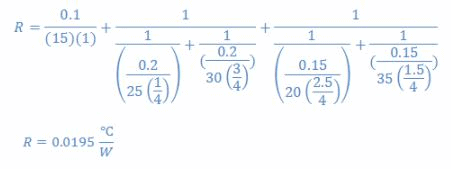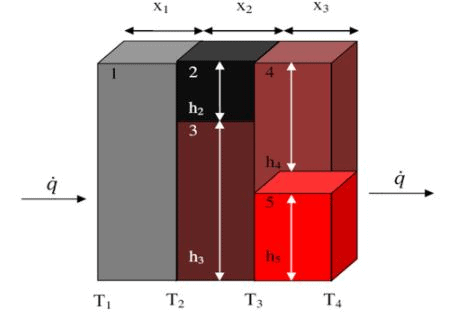Fig. 2.7: Composite of illustration 2.3; (a) composite, (b) corresponding electrical circuit

Thus the heat flow rate through the composite,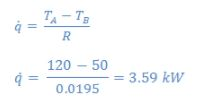2.2 Thermal contact resistance
In the previous discussion, it was assumed that the different layers of the composite have perfect contact between any two layers. Therefore, the temperatures of the layers were taken same at the plane of contact. However, in reality it rarely happens, and the contacting surfaces are not in perfect contact or touch as shown in the fig.2.8(a). It is because as we know that due to the roughness of the surface, the solid surfaces are not perfectly smooth. Thus when the solid surfaces are contacted the discrete points of the surfaces are in contact and the voids are generally filled with the air. Therefore, the heat transfer across the composite is due to the parallel effect of conduction at solid contact points and by convection or probably by radiation (for high temperature) through the entrapped air. Thus an apparent temperature drop may be assumed to occur between the two solid surfaces as shown in the fig.2.8b. If TI and TII are the theoretical temperature of the plane interface, then the thermal contact resistance may be defined as,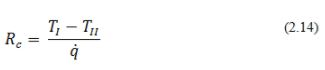where Rcrepresents the thermal contact resistance.

The utility of the thermal contact resistance (Rc ) is dependent upon the availability of the reliable data. The value of Rc depends upon the solids involved, the roughness factor, contact pressure, material occupying the void spaces, and temperature.  The surface roughness of a properly smooth metallic surface is in the order of micrometer. The values of Rc generally obtained by the experiments. However, there are certain theories which predict the effect of the various parameters on the Rc.

It can be seen in the fig.2.8, that the two main contributors to the heat transfer are (i) the conduction through entrapped gases in the void spaces and, (ii) the solid-solid conduction at the contact points. It may be noted that due to main contribution to the resistance will be through first factor because of low thermal conductivity of the gas.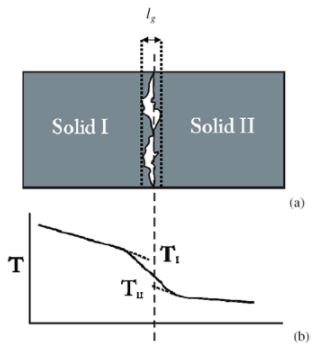Fig.2.8 (a) Contacting surfaces of two solids are not in perfect contact, (b) temperature drop due to imperfect contact

If we denote the void area in the joint by Av and contact area at the joint by Ac, then we may write heat flow across the joint as,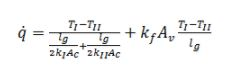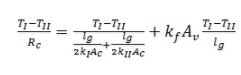where, thickness of the void space and thermal conductivity of the fluid (or gas) is represented by lgand kf, respectively. It was assumed that lg/2 is the thickness of solid-I and solid-II for evenly rough surfaces.

Offer running on EduRev: Apply code STAYHOME200 to get INR 200 off on our premium plan EduRev Infinity!

## Heat Transfer for Engg.

46 videos|60 docs|59 tests

,

,

,

,

,

,

,

,

,

,

,

,

,

,

,

,

,

,

,

,

,

;